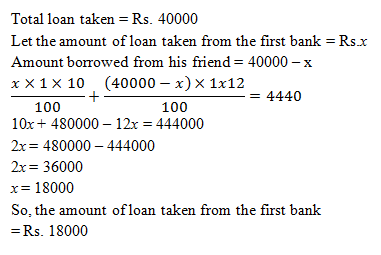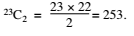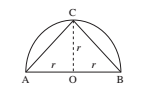# SSC CGL 2018 Practice Test Papers | Quantitative Aptitude (Day-31)

Dear Aspirants, Here we have given the Important SSC CGL Exam 2018 Practice Test Papers. Candidates those who are preparing for SSC CGL 2018 can practice these questions to get more confidence to Crack SSC CGL 2018 Examination.

[WpProQuiz 3209]

Click “Start Quiz” to attend these Questions and view Explanation

1) A rectangular garden is 120 m × 80 m. There is a path along the garden and just outside it. Width of the path is 12 m. The area of the path is –

a) 4389

b) 6000

c) 3264

d) 5376

2) Amrish takes a loan of Rs. 40000 partly from a bank at 10% per annum and remaining from his friend at 12% per annum. He pays total interest of Rs. 4440 per annum. Amount of loan taken from the bank is –

a) Rs. 22000

b) Rs. 20000

c) Rs. 18000

d) Rs. 16000

3) If x3 + 5x2 + 10k leaves remainder – 2x when divided by x2 + 2, then what is the value of k ?

a) –2

b) –1

c) 1

d) 2

4) A tradesman sold an article at a loss at 25%. If the selling price had been increased by Rs. 210 there would have been a gain of 10%. The cost price of article is –

a) Rs. 300

b) Rs. 450

c) Rs. 571

d) Rs. 600

5) A man covers 3/14 of the total journey by train, 7/18 by bus and the remaining 30 km on foot. His total journey is

a) 24 km

b) 32 km

c) 48 km

d) 75.6 km

6) A two digit number is such that when it is divided by the largest single digit prime number leaves remainder 4. If the difference of the two digits is 4, then the number is—

a) 73

b) 25

c) 53

d) 95

7)  The number of straight lines that can be drawn in a plane with 23 given points, assuming that no three of them are collinear is—

a) 253

b) 46

c) 2·23!

d) 21!

8) Area of the largest triangle drawn in a semicircle of radius r will be—

a) r2

b) r3

c) 2r2

d) 2r3

9) What is the value of (tan460° – sin490°) – 2(tan245° – 3cos0°)2?

a) 0

b) 1

c) 3

d) 4

10) Simplify: 25×√15625×2+3=?

a) 6253

b) 3450

c) 1563

d) 1560

The area of rectangular garden with path

= (120 + 24) × (80 + 24)

= 144 × 104

= 14976 m2

The area of rectangular garden = 120 × 80

= 9600 m2

So, area of path = 14976 – 9600 = 5376 m2If an equation is divisible by (x2+ 2) then substituting x2= – 2 in that equation must give 0 as a result.

(x3 + 5x2 + 10k + 2x) is divisible by x2 + 2.

x3 + 2x + 5x2 + 10k = 0

x(x2 + 2) + 5(x2 + 2) + 10k – 10 = 0

0 + 0 + 10k – 10 = 0

K=10/10=1

CP                           SP

100  ————— 75(25% loss)

100  —————  110(10 % profit)

If selling price is increased by Rs.35, then cost price = Rs. 100

Originally selling price is increased by Rs. 210, so cost price = Rs. 100 /35 x210 = Rs. 600

Let total journey =  x km

x-(3x/14)-(7x/18)=30

x(126-27-49)/126=30

x=75.6 km

Largest single digit prime number = 7

On dividing 95 by 7 remainder is 4 and the difference of two digits is (9 – 5) = 4Perpendicular height of the largest triangle drawn in a semi-circle of radius r = r∴ Area of the largest possible triangle = 1/ 2 (base × height) = 1/ 2 (2r) × (r) = r2

(tan460° – sin490°) – 2(tan245° – 3cos0°)2

= [(√3 )4 – (1)4] – 2(1 – 3)2

= (9 – 1) – 2(4)

= 8 – 8 = 0